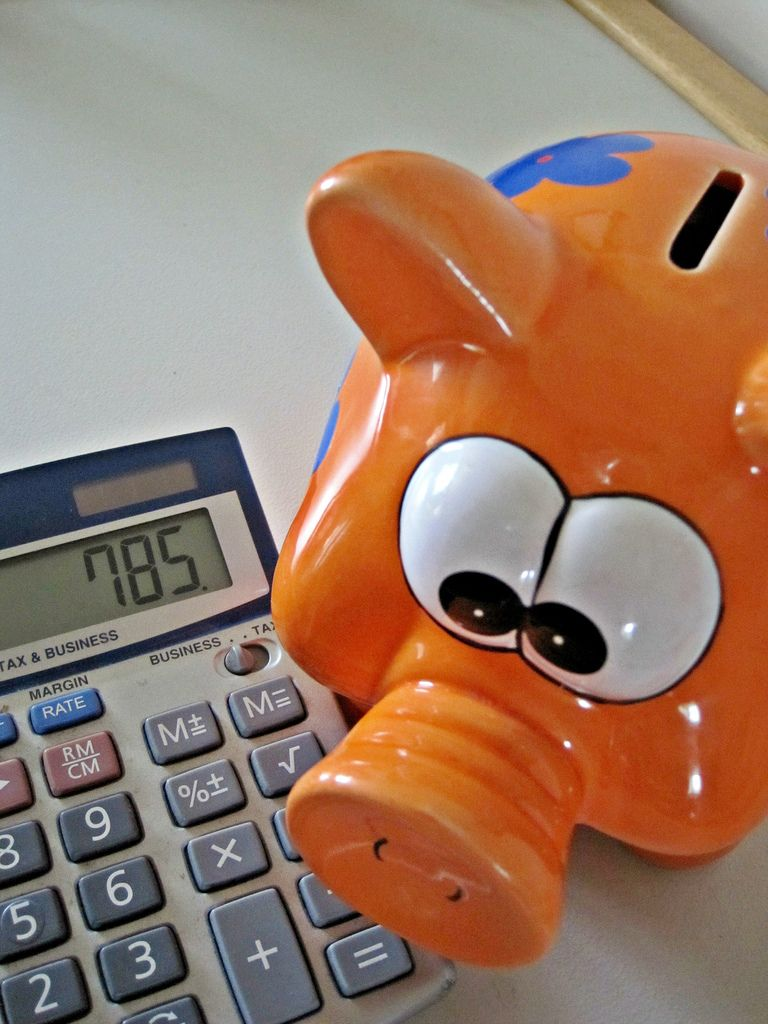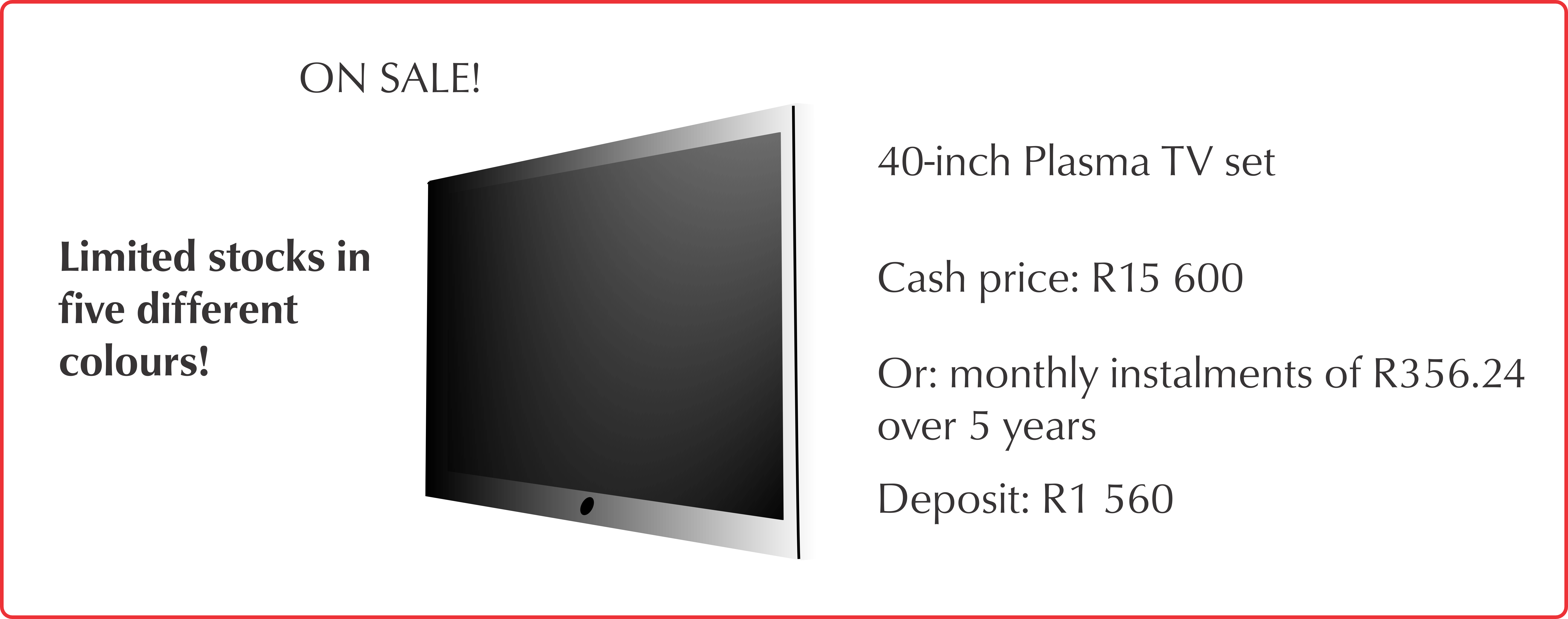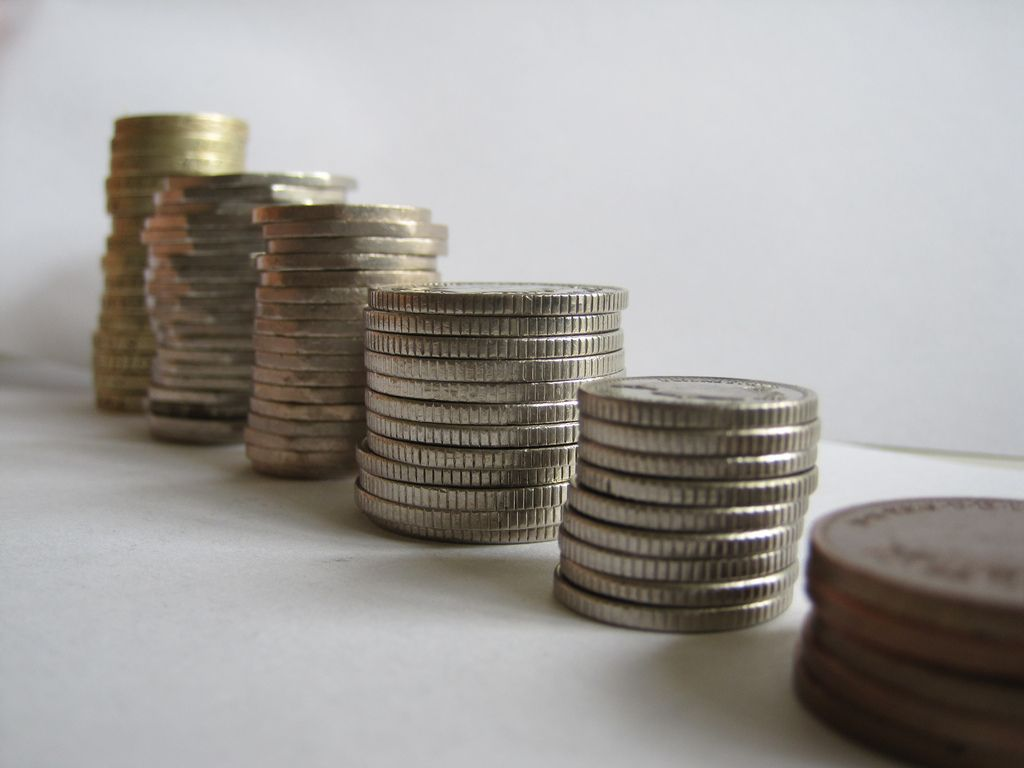Home Practice
For learners and parents For teachers and schools
Textbooks
Full catalogue
Pricing SupportLog in

We think you are located in United States. Is this correct?

# 11.3 Interest

## 11.3 Interest (EMG6H)

People need to pay interest when they borrow money, either from a bank, or if they purchase an item from a shop and pay it off in monthly installments using a hire purchase option. Buying an item on hire purchase is also borrowing money. The interest amount is calculated as a percentage of the amount owing.

If you save money in a bank account, you also earn interest on the balance in your account.Interest rate
A percentage charged for the borrowing, or loan, of a sum of money over a given period of time.
Interest
The amount of money that you are charged (by the lender of money, e.g. the bank) for borrowing an amount of money, over a period of time.

### Interest rates (EMG6J)

Interest rates can vary considerably. The interest rate that a person is charged depends on how much money they leave invested in the bank, and on other factors, including:

• The South African Reserve Bank's lending rate (repo-rate) to financial institutions. This can change from month to month, and banks usually link their rates to the repo-rate.
• Different banks may offer different deals, depending on various conditions. For example, you may get a lower interest rate on a loan if you open an account with the same bank.
• Credit ratings: If you are opening your first bank account you do not have a “banking history”. This means you will not have a credit rating history either and will probably be charged a higher interest rate if you wanted to take out a loan.
• How long you have been employed in your present job or whether you own assets such as a home.
• If you save more money for a longer period, the bank is likely to offer a higher interest rate on your savings.

### Calculating interest amounts and interest rates (EMG6K)

If we know what the interest rate is, we can calculate the interest value quite simply:

$$\text{10}\%$$ interest on $$\text{R}\,\text{3 500}$$ = $$\text{R}\,\text{3 500}$$ $$\times$$ $$\text{10}\%$$ = $$\text{R}\,\text{350}$$. So the interest amount is $$\text{R}\,\text{350}$$ and the total amount is $$\text{R}\,\text{3 500}$$ + $$\text{R}\,\text{350}$$ = $$\text{R}\,\text{3 850}$$.

If you are given the final amount, then you follow these steps to find the interest rate:

• Find the difference between the final amount and the original amount: this gives you the amount of interest.
• Work out what percentage the amount of interest is of the original value.

Let's look at a worked example to see how to do this.

## Worked example 4: Understanding interest

Look at the advert below. You can buy a 3-piece wall unit cash for $$\text{R}\,\text{6 499,99}$$. Alternatively, you could choose to buy it on hire purchase and pay for it in installments over $$\text{3}$$ years. If you choose to pay it off in installments, you will pay interest every month on the wall unit.1. Calculate what the wall unit will cost if you pay a cash deposit of $$\text{R}\,\text{650}$$, and $$\text{36}$$ monthly installments of $$\text{R}\,\text{449}$$ each. (Total = cash deposit + $$\text{36}$$ monthly installments).
2. Calculate how much interest you will pay in total (in Rands) if you pay off the wall unit in installments. (Hint: interest amount = total payments - cash price).
3. Calculate the interest rate. (Interest rate = (Interest $$\div$$ total paid) $$\times$$ $$\text{100}$$).
4. Do you think it is better to save up and buy the wall unit at the cash price, or pay it off over $$\text{3}$$ years? Explain your answer.
1. Total = cash deposit + $$\text{36}$$ monthly installments = $$\text{R}\,\text{650}$$ + ($$\text{R}\,\text{449}$$ $$\times$$ $$\text{36}$$ months) = $$\text{R}\,\text{650}$$ + $$\text{R}\,\text{16 164}$$ = $$\text{R}\,\text{16 814}$$
2. Interest = Installment total - cash price = $$\text{R}\,\text{16 814}$$ - $$\text{R}\,\text{6 499,99}$$ = $$\text{R}\,\text{10 314,01}$$.
3. Interest rate = $$\frac{\text{interest amount}}{\text{total amount}} \times \text{100} = \frac{\text{ R}\text{10 314,01}}{\text{R}\,\text{16 814,00}} \times \text{100} = \text{0,613} \times \text{100} = \text{61,4}\%$$.
4. It is much cheaper to save up and buy the wall unit at the cash price. Over three years, the total amount you would pay in installments is more than twice as expensive as the cash price!

## Understanding interest

Exercise 11.3Does the advertisement indicate the percentage of interest that will be charged if the TV is not paid for in cash?

No.

What will the balance be once the deposit has been paid?

Balance = Cash Price - Deposit = $$\text{R}\,\text{15 600}$$ - $$\text{R}\,\text{1 560}$$ = $$\text{R}\,\text{14 040}$$

Will the interest be charged on the full purchase price or on the balance?

It will be charged on the account balance.

How much will the installments be per month?

$$\text{R}\,\text{356,24}$$

How much will you have to pay for the TV in total? Use the formula: Total to be paid = Deposit + (Installment amount $$\times$$ number of installments)

Total Payable = Deposit + (Installment amount $$\times$$ number of installments) =$$\text{1 560}$$ + ($$\text{356,24}$$ $$\times$$ [($$\text{12}$$ $$\times$$ $$\text{5}$$)]=$$\text{R}\,\text{1 560}$$ + $$\text{R}\,\text{21 374,40}$$ = $$\text{R}\,\text{22 934,40}$$

How much interest (in Rands) will you have paid once you have completed paying off the TV ? Use the formula: $$\frac{\text{Total interest payable}}{\text{Value}}$$ = (Installment amount $$\times$$ number of installments) $$-$$ Balance

$$\frac{\text{Total interest payable}}{\text{Value}}$$ = (Installment amount $$\times$$ number of installments) $$-$$ Balance = $$\text{R}\,\text{356,24}$$ $$\times$$ [ $$\text{12}$$ $$\times$$ $$\text{5}$$ ] $$-$$ $$\text{14 040}$$ = $$\text{R}\,\text{21 374,40}$$ $$-$$ $$\text{R}\,\text{14 040}$$ = $$\text{R}\,\text{7 334,40}$$

## Worked example 5: Understanding interest

Grant borrows $$\text{R}\,\text{15 000}$$ from his friend, Molefe, to finish an order for his customers. Molefe offers the following:

• The loan plus $$\text{12}\%$$ simple interest per annum
• Interest Value = Interest rate as a percentage of the borrowed amount.
• Total Repayment = borrowed amount + interest value.
1. Calculate the amount of interest for the interest rate Molefe offered.
2. How much in total will Grant have to pay?1. Interest amount = $$\frac{\text{12}}{\text{100}} \times$$ $$\text{R}\,\text{15 000}$$ = $$\text{R}\,\text{1 800}$$
2. Total repayment = Borrowed Amount + Interest Value. = $$\text{R}\,\text{15 000}$$ + $$\text{R}\,\text{1 800}$$ = $$\text{R}\,\text{16 800}$$.